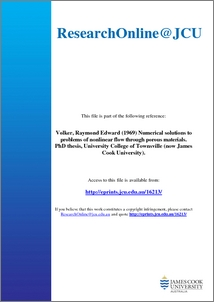# Numerical solutions to problems of nonlinear flow through porous materials

Volker, Raymond Edward (1969) Numerical solutions to problems of nonlinear flow through porous materials. PhD thesis, University College of Townsville (now James Cook University).Preview
PDF (Thesis)

## Abstract

Two commonly suggested forms of the equation linking head loss and velocity for flow of water through coarse granular media are the Forchheimer and exponential relations. These have been combined with the continuity expression to give the differential equations applicable, within the limits of validity of the parent relations, to actual regions of flow. The resultant nonlinear elliptic partial differential equations have been solved by numerical methods including the direct finite difference and finite element methods.

Experimental results and associated analytical work were carried out to determine the accuracy of the nonlinear relations as compared to the linear Darcy Law, when applied over an extended Reynolds number range. Solutions have been obtained for some examples of unconfined flow with boundary conditions similar to those likely to be encountered in practical applications. The experimental work in a circular tank and an open flume has shown that good agreement between observed and calculated values of discharge and piezometric head can be obtained when the coefficients in the nonlinear head loss equations are accurately known. The results indicate that while the flow patterns from the Darcy and the nonlinear solutions are only significantly different for a high degree of curvature of the phreatic line, a nonlinear solution will usually be necessary for accurate predictions of discharge.

Item ID: 16213 Thesis (PhD) nonlinear flow, porous materials, head loss, velocity, flow patterns, flow conditions, aquifers, Forchheimer relation, finite difference, finite element methods, Darcy's Law, field equations, numerical solutions Raymond Volker received a JCU Outstanding Alumni Award in 2010. 30 Mar 2011 00:31 09 ENGINEERING > 0905 Civil Engineering > 090509 Water Resources Engineering @ 40%01 MATHEMATICAL SCIENCES > 0103 Numerical and Computational Mathematics > 010302 Numerical Solution of Differential and Integral Equations @ 30%09 ENGINEERING > 0912 Materials Engineering > 091299 Materials Engineering not elsewhere classified @ 30% 97 EXPANDING KNOWLEDGE > 970109 Expanding Knowledge in Engineering @ 60%97 EXPANDING KNOWLEDGE > 970101 Expanding Knowledge in the Mathematical Sciences @ 40% Total: 2359 Last 12 Months: 20 More Statistics

Actions (Repository Staff Only)Item Control Page